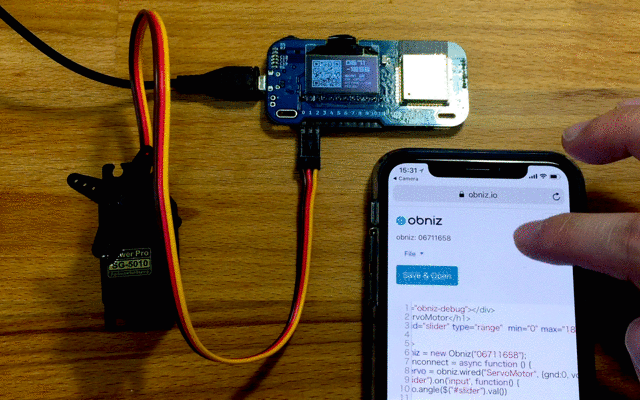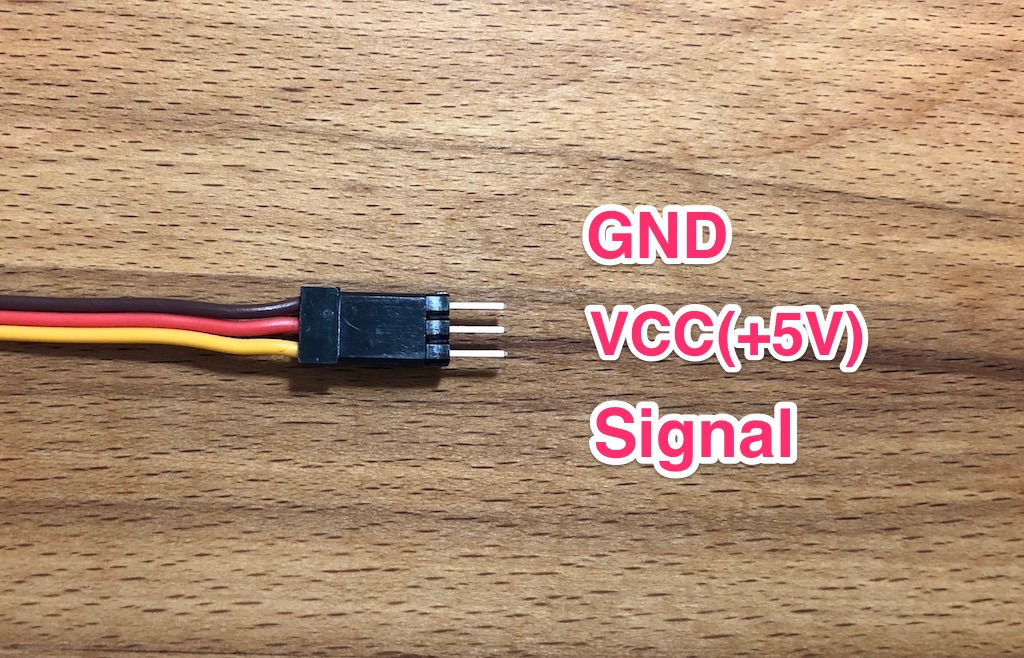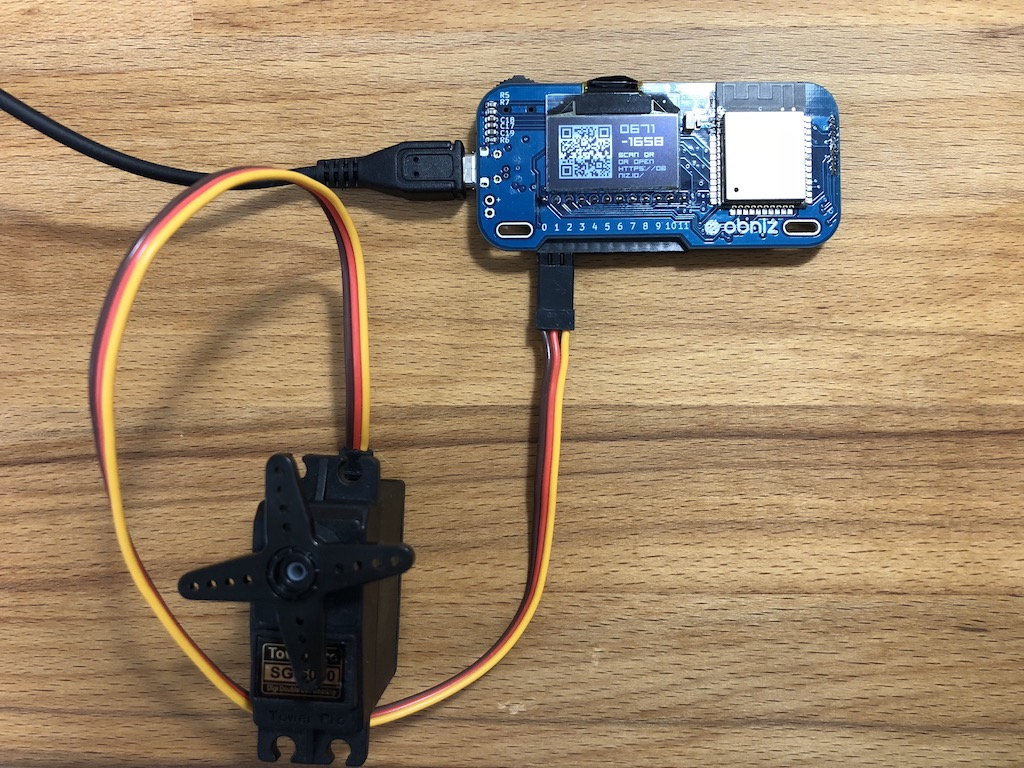# Servo Motor

In this lesson, we will use RC servo motor.## Connect

Servo motor is listed on the SDK Parts library, the details of which can be seen here: ServoMotor.

First, connect a servomotor to obniz.
RC servo motor usually has 3 wires - vcc(+), gnd(-), and Signal(angle control)Let's connect as below

1. io0 servomotor gnd
2. io1 servomotor vcc
3. io2 servomotor signal

It should look ike this## Slider

Wouldn't it be super cool if a servo can be controlled from HTML slider!?
The slider looks like this. You can slide it with your mouse or finger.

The slider is indicated by `<input type="range">`.

``````<input id="slider" type="range"  min="0" max="180" />
``````

As noted, the slider is defined by `input type="range"`.
Here, the id is "slider". (but you can choose whatever you want to be the id. "slider" is just an example.)
min and max define the value range. We want to control the range of the servomotor within 0 and 180 degrees, so `min=0` and `max=180`.

Next, we will see how to control changing positions of the slider.

``````\$("#slider").on('input', function() {
// this function will be called when someone move a slider.
var val = \$("#slider").val();
});
``````

The program below allows you to know exactly how much the slider has been moved. `\$("#slider").val()` gets you the current value of the slider position, which is between 0 and 180.

## Control Servomotor

See the details here: ServoMotor
To change the angle of a servomotor, the necessary program is shown below.

``````var servo = obniz.wired("ServoMotor", {gnd:0, vcc:1, signal:2});

servo.angle(90.0); // half position
``````

`servo.angle(90)` means it will change the motor's angle to 90 degrees.
The value must be between 0 and 180.
This is why it was set so that min is 0 and max is 180!

The full code is shown below.

``````<!-- HTML Example -->
<html>
<meta name="viewport" content="width=device-width, initial-scale=1">
<script src="https://obniz.com/js/jquery-3.2.1.min.js"></script>
<script src="https://unpkg.com/obniz@3.3.0/obniz.js"></script>
<body>

<div id="obniz-debug"></div>
<h1>ServoMotor</h1>
<input id="slider" type="range"  min="0" max="180" />

<script>
var obniz = new Obniz("OBNIZ_ID_HERE");
obniz.onconnect = async function () {
var servo = obniz.wired("ServoMotor", {gnd:0, vcc:1, signal:2});
\$("#slider").on('input', function() {
servo.angle(\$("#slider").val())
});
};

obniz.onclose = async function(){
\$("#slider").off('input');
};
</script>
</body>
</html>
``````

Copy&paste this and put in your obniz id.
If you run this program, you will see the slider on HTML.
Try moving the slider, and you should see your servo motor change its angle.